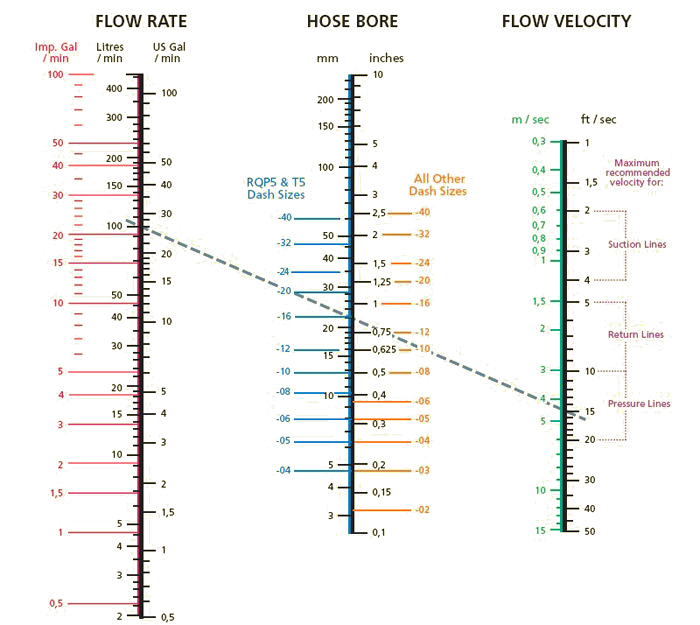# Nomograph

### What is a Nomograph?

In a nutshell, a Nomograph is a graph, usually containing three parallel scales graduated for different variables so that when a straight line connects values of any two, the related value may be read directly from the third at the point intersected by the line.

Inexpensive scientific and engineering calculators have pretty much eliminated the need for nomographs that embody complicated relationships that would prove cumbersome or difficult to calculate otherwise.

A basic nomograph used to calculate reactance includes logarithmic scales for inductance, capacitance, reactance and frequency, as shown below. In this example, the straight line, called an isopleth, connects the 10 cycles/second (cps) to a 5-Henry inductance. To obtain the 10 cps resonance, you will need a 50 μF capacitor. The nomograph also lets you determine the XL or XC reactance, too.1. Pick the two known values.
2. Lay a straightedge to intersect the two values.
3. Intersection on the third vertical line gives the value of that factor.

Nomograph Example:
To find the bore size for a Pressure Line consistent with a Flow Rate of 100 litres per minute (26 US or 22 Imperial gallons per minute), and a Flow Velocity of 4,5 metres per second (14.8 feet per second), connect Flow Rate to Flow Velocity and read Hose Bore on centre scale.